Next: Summary Up: Labels Previous: Choosing names for

### When to use labels

The simple answer is to use a label whenever it will make it easier for you to do so. Of course, being able to decide this depends mainly on how much experience you have with Maple, so it isn't always easy to do this at first.

In general, you should label the result of a command if you will need to use that result in a later computation. If you won't need the result later, there is no need to label it.

One of the strengths of a CAS, like Maple, is the ability to go back and change or correct a series of computations. Using labels can make this process easier. For example, suppose you were given some data pairs and wanted to fit a polynomial to the data. Consider the following data on tool wear in a machining process.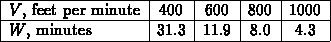The independent variable is V, the cutting speed in feet per minute and the dependent variable is W, the cutting time, in minutes, for the tool to wear out. Suppose you needed to estimate values of W for values of V not in the table. One way to do this is to fit the data to a polynomial. In this case, a natural choice for a polynomial is a cubic, because there are 4 data points and 4 coefficients in a cubic polynomial. A simple Maple command for doing this is shown below.

```  > interp([400,600,800,1000],[31.3,11.9,8.0,4.3],V);
```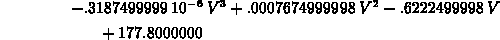If this is all you need the data points for, this approach is just fine. Suppose, however, that you wanted to fit the same data with several different functions and/or you wanted to change the data slightly and fit it again . Then it might make sense to label the data points as shown below.

```  > V_vals := [400,600,800,1000];
```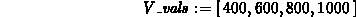```  > W_vals := [31.3,11.9,8.0,4.3];
```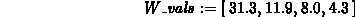```  > interp(V_vals,W_vals,V);
```For a single fit, this is more work, but suppose you wanted to fit the data to an expression of the forminstead of a simple polynomial. This can be done using the labels defined above and the following command.

```  > subs(x=1/V,interp(map(s->1/s,V_vals),W_vals,x));
```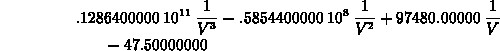This command looks rather complicated, but it was written to get around the fact that the interp command will only do polynomials. This is an example of a compound command, which can be extremely useful in solving problems with Maple. A description of how to build up compand commands appears later in this document, and the command given above will be used as an example there.

Now, suppose you wanted to change the data set slightly and generate new interpolating expressions. To do this, you would only have to go back and change `V_vals` and/or `W_vals` and then reissue all of the commands. If you didn't use labels, you would have to change the list entries for each of the interpolating expressions, leading to more chances for typing errors.

It is also probably a good idea to label the two interpolating expressions, so they can be plotted or otherwise used. It is not necessary to retype their definitions again. Simply go back and edit the commands, adding labels as shown below.

```  > p1 := interp(V_vals,W_vals,V);
```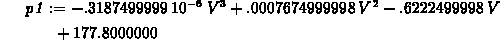```  > p2 := subs(x=1/V,interp(map(s->1/s,V_vals),W_vals,x));
```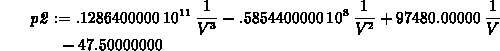```  > plot(p1,p2,V=400..1000);
```

```  > plot(p1-p2,V=400..1000);
```

The two plot commands at the end allow you to compare the two interpolating functions. Notice that they both go through the data points, but can differ significantly away from them.

It would also have been possible to make the interpolating expressions into functions. Whether you would want to do so or not depends on how you wanted to use them later on in the worksheet. When to use functions rather than expressions is the subject of the next section of this document.Next: Summary Up: Labels Previous: Choosing names for

William W. Farr
Mon Aug 28 09:31:56 EDT 1995